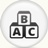# Verbal Reasoning - Alphabet SeriesVerbal Reasoning – Alphabet Series Aptitude Questions and Answers with solutions and Explanations for Bank PO, UPSC, CSAT, MBA, CAT, SSC-CGL, IBPS, Bank Clerical etc.

# Verbal Reasoning →Series Completion → Alphabet Series: Question – 40

Find the missing term in the following series: ejo, tyd, ins, xch, ? [A]nrw [B]mrw [C]msx [D]nsx Show Answer mrw In the above question, the pattern is as follows: There is a gap of four letters between the first and second, the second and third letters of each term, and also between the last letter ..

# Verbal Reasoning →Series Completion → Alphabet Series: Question – 39

Find the missing term in the following series: AYD, BVF, DRH, ?, KGL [A]FMI [B]GMJ [C]GLJ [D]HLK Show Answer GMJ In the above question, the pattern is, 1st letter $latex A\xrightarrow{\text{+1}}B \xrightarrow{\text{+2}}D \xrightarrow{\text{+3}}G \xrightarrow{\text{+4}} \textcircled{K}$ 2nd term $latex Y\xrightarrow{\text{-3}}V \xrightarrow{\text{-4}}R \xrightarrow{\text{-5}}M \xrightarrow{\text{-6}} \textcircled{G}$ 3rd term $latex D\xrightarrow{\text{+2}}F \xrightarrow{\text{+2}}H \xrightarrow{\text{+2}}J \xrightarrow{\text{+2}} \textcircled{L}$

# Verbal Reasoning →Series Completion → Alphabet Series: Question – 38

Find the missing term in the following series: DEF, HIJ, MNO, ? [A]STU [B]RST [C]RTV [D]SRQ Show Answer STU In the above question, the pattern is, 1st letter $latex D\xrightarrow{\text{+4}} H \xrightarrow{\text{+5}} M \xrightarrow{\text{+6}}\textcircled{S}$ 2nd letter $latex E\xrightarrow{\text{+4}} I \xrightarrow{\text{+5}} N \xrightarrow{\text{+6}}\textcircled{T}$ 3rd letter $latex F\xrightarrow{\text{+4}}J \xrightarrow{\text{+5}} O \xrightarrow{\text{+6}}\textcircled{U}$

# Verbal Reasoning →Series Completion → Alphabet Series: Question – 37

Find the missing term in the following series: UPI, ?, ODP, MBQ, IAW [A]RHJ [B]SHJ [C]SIJ [D]THK Show Answer SHJ In the above question, the pattern is, 1st letter $latex Y\xrightarrow{\text{-2}}\textcircled{S} \xrightarrow{\text{-4}}O \xrightarrow{\text{-2}}M \xrightarrow{\text{-4}}I$ 2nd letter $latex P\xrightarrow{\text{-8}}\textcircled{H} \xrightarrow{\text{-4}}D \xrightarrow{\text{-2}}B \xrightarrow{\text{-1}}A$ 3rd letter $latex I\xrightarrow{\text{+1}}\textcircled{J} \xrightarrow{\text{+6}}P \xrightarrow{\text{+1}}Q \xrightarrow{\text{+6}}W$

# Verbal Reasoning →Series Completion → Alphabet Series: Question – 36

Find the missing term in the following series: AZY, BUT, CXW, DWV, ? [A]EVA [B]EVU [C]VEU [D]VUE Show Answer EVU In the above question, the pattern is, 1st letter $latex A\xrightarrow{\text{+1}}B\xrightarrow{\text{+1}}C \xrightarrow{\text{+1}} D\xrightarrow{\text{+1}} \textcircled{E}$ 2nd letter \$latex Z\xrightarrow{\text{-5}}U\xrightarrow{\text{+ (5 – 2) + 3}}X \xrightarrow{\text{- (3 – 2 =) – 1}} W\xrightarrow{\text{+(1 – 2 ) ..